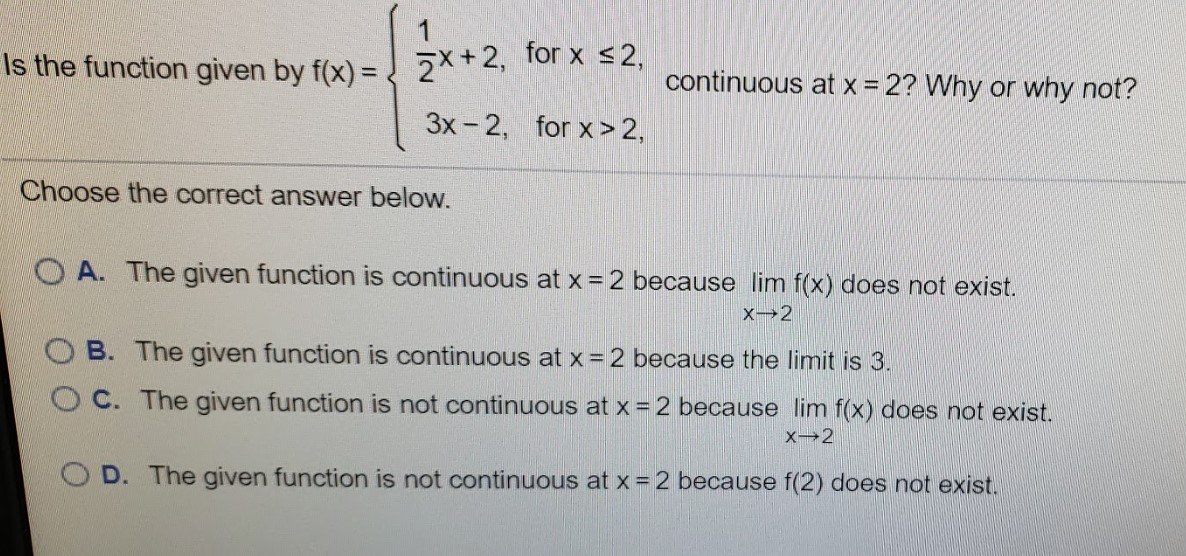# 2*+2, for x 2,Choose the correct answer below.O A. The given function is continuous at x = 2 because lim f(x) does not exist.O B. The given function is continuous at x = 2 because the limit is 3.OC. The given function is not continuous at x = 2 because lim f(x) does not exist.OD. The given function is not continuous at x =2 because f(2) does not exist.

Question
4 viewshelp_outlineImage Transcriptionclose2*+2, for x <2, Is the function given by f(x) = continuous at x = 2? Why or why not? %3! 3x - 2, for x> 2, Choose the correct answer below. O A. The given function is continuous at x = 2 because lim f(x) does not exist. O B. The given function is continuous at x = 2 because the limit is 3. OC. The given function is not continuous at x = 2 because lim f(x) does not exist. OD. The given function is not continuous at x =2 because f(2) does not exist. fullscreen
check_circle

star
star
star
star
star
1 Rating
Step 1

The function y = f(x) is continuous at point x = a if the following three conditions are satisfied.

Step 2

Now, determine the values of the...

### Want to see the full answer?

See Solution

#### Want to see this answer and more?

Solutions are written by subject experts who are available 24/7. Questions are typically answered within 1 hour.*

See Solution
*Response times may vary by subject and question.
Tagged in

### Calculus